Next: Degenerate parabolic equations Up: Regularizing effect revisited () Previous: Regularizing effect revisited ()

## Kinetic and other approximations

Theorem 2.3.1 provides an alternative route to analyze the convergence of general entropy stable multi-dimensional schemes, schemes whose convergence proof was previously accomplished by measure-valued arguments; here we refer to finite-difference, finite-volume, streamline-diffusion and spectral approximations ..., which were studied in [4, 18, 19, 15, 16, 3]. Indeed, the feature in the convergence proof of all these methods is the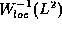-compact entropy production, (2.4.33). Hence, if the underlying conservation law satisfies the non-linear degeneracy condition (2.3.22), then the corresponding family of approximate solutions,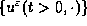becomes compact. Moreover, if the entropy production is bounded measure, then there is actually a gain of regularity indicated in Theorem 2.3.1 and respectively, in (2.3.21) for the translation invariant case. Remark. Note that unlike the requirement for a nonpositive entropy production from measure-valued solutions (consult () in Lecture I), here we allow for an arbitrary bounded measure.

So far we have not addressed explicitly a kinetic formulation of the multidimensional conservation law (2.3.15). The study of regularizing effect for multidimensional conservation laws was originally carried out in  for the approximate solution constructed by the following BGK-like model,  (see also ,),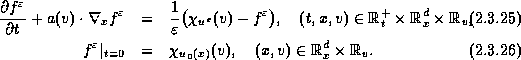Here,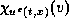denotes the `pseudo-Maxwellian',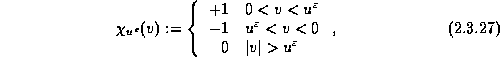which is associated with the average of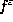,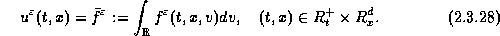The key property of this kinetic approximation is the existence of a nonnegative measure,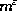such that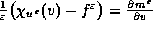(The existence of such measures proved in  and is related to H-functions studied in  and Brenier's lemma .) Thus, we may rewrite (2.3.25) in the form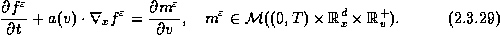Let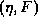be an entropy pair associated with (2.3.15). Integration of (2.3.29) against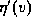implies that the corresponding macroscopic averages,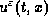, satisfy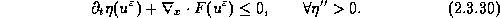Thus, the entropy production in this case is nonpositive and hence a bounded measure, so that Theorem 2.3.1 applies. Viewed as a measure-valued solution, convergence follows along DiPerna's theory . If, moreover, the nondegeneracy condition (2.3.16) holds, then we can further quantify the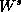-regularity (of order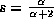.)

Theorem 2.3.1 offers a further generalization beyond the original, 'kineticly' motivated discussion in . Indeed, consideration of Theorem 2.3.1 reveals the intimate connection between the macroscopic assumption of bounded entropy production in (2.3.17), and an underlying kinetic formulation ({2.3.20), analogous to (2.3.29). For a recent application of the regularizing effect for a convergence study of finite-volume schemes along these lines we refer to .Next: Degenerate parabolic equations Up: Regularizing effect revisited () Previous: Regularizing effect revisited ()